## 20 Questions MCQ Test NCERT Mathematics for CAT Preparation | Olympiad Test: Rational Numbers

Description
Attempt Olympiad Test: Rational Numbers | 20 questions in 20 minutes | Mock test for Class 8 preparation | Free important questions MCQ to study NCERT Mathematics for CAT Preparation for Class 8 Exam | Download free PDF with solutions
QUESTION: 1

### Which of the following statements is true?

Solution:

Every rational number is not a fraction. In rational numbers, we use integers and in fractions, we use only natural numbers.

QUESTION: 2

### Compare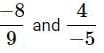Solution:

Expressing in standard form, we get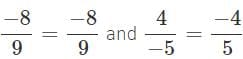Express each rational number with L.C.M as denominator. L.C.M of 9 and 5 is 9 × 5 = 45.

∴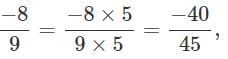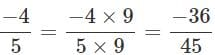Comparing the numerators, we have −40<−36 .

∴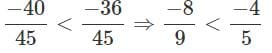QUESTION: 3

### How is -28/84 expressed as a rational number with numerator 4?

Solution:

Given, -28/84
Dividing both the numbers by 7.
Therefore, -4/12.

QUESTION: 4

17/11 - 6/11

Solution: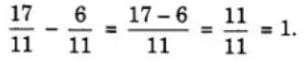QUESTION: 5

Which of the following statements is false?

Solution: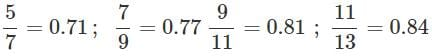So,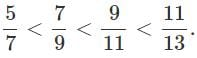Hence, both (b) and (c) are false.

QUESTION: 6

Which of the following is a rational number between 1/4 and 1/3 ?

Solution: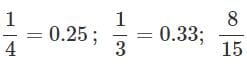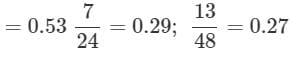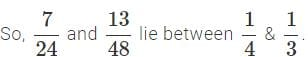QUESTION: 7

Which of the following is the correct arrangement of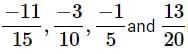in descending order?

Solution:

LCM of 5,10,15 and 20 which is 60. Now making the denominator same, we have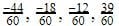. So descending order is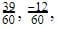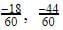that is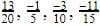QUESTION: 8

Simplify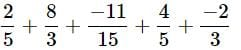Solution:

LCM of 5,3 and 15 is 15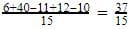QUESTION: 9

What is the sum of the additive inverse and multiplicative inverse of 2?

Solution:

Additive inverse of 2 is −2
Multiplicative inverse of 2 is 1/2
∴ The sum of multiplicative inverse and additive inverse =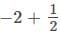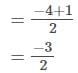Answer :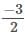QUESTION: 10

Subtract 2/3 from 7/8

Solution:

Solution :- 7/8 - 2/3

= {(21-16)/24}

= 5/24

QUESTION: 11

Which among the following is a rational number equivalent to -5/-3 ?

Solution: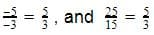Hence the correct answer is 5/3.

QUESTION: 12

The value of (5/4) – (8/3) is:

Solution:

5/4 – 8/3

Making the denominator equal:

[(5/4) x (3/3)] – [(8/3) x (4/4)]

= (15/12) – (32/12)

= (15 – 32)/12

= -17/12

QUESTION: 13

Which number is in the middle if -1/6, 4/9, 6/-7, 2/5 and -3/4 arranged in descending order?

Solution:

-⅙ = -0.16

4/9 = 0.44

-6/7 = -0.85

⅖ = 0.40

-¾ = -0.75

Descending Order : - 4/9, 2/5, -1/6, -3/4, -6/7

Middle term : - -1/6

QUESTION: 14

What is the average of the two middle rational numbers when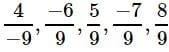and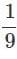are arranged in ascending order?

Solution:

Arranging the given rational numbers in ascending order, we  get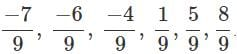The two middle rational numbers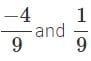Their average is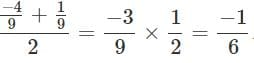QUESTION: 15

Subtract -5/9 from -3/7.

Solution:

(-3*9 + 5*7 )/63

8/63

QUESTION: 16

What number should be added to -4/7 to get 5/9 ?

Solution:

Let the number be x

Then,

X+(-4/7)=5/9

X=5/9+4/7

X= 35+36/63

X=71/63

QUESTION: 17

What should be subtracted from -7/11 to get −2?

Solution:

Let x be subtracted from -7/11
then -7/11 - x= -2
-x= -2 + (7/11)
-x= -2 + 7/11
-x= -15/11
x= 15/11

QUESTION: 18

By what rational number should -3/5 be multiplied to obtain 21?

Solution:

Let the rational no be x
-3/5 X x = 21
x = (21 X5 )/ -3
x = -35

QUESTION: 19

The sum of two rational numbers is −3. If one of them is -11/5, find the other.

Solution:

Let the other number be x

x+(-11/5)= -3

x= -3+11/5

x=-15+11/5

x= -4/5

QUESTION: 20

Which of the following is the resultant of 1/12 + -1/3 + -1/6 + -5/7 + 3/4 + 10/7?

Solution: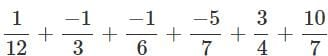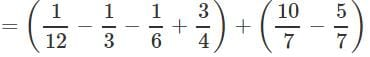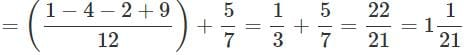Use Code STAYHOME200 and get INR 200 additional OFF Use Coupon Code